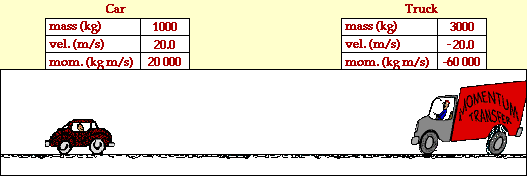## Car and Truck in Head-on Collision

### Inelastic Collision

Collisions between objects are governed by laws of momentum and energy. When a collision occurs in an isolated system, the total momentum of the system of objects is conserved. Provided that there are no net external forces acting upon the objects, the momentum of all objects before the collision equals the momentum of all objects after the collision. If there are only two objects involved in the collision, then the momentum change of the individual objects are equal in magnitude and opposite in direction.

Certain collisions are referred to as elastic collisions. Elastic collisions are collisions in which both momentum and kinetic energy are conserved. The total system kinetic energy before the collision equals the total system kinetic energy after the collision. If total kinetic energy is not conserved, then the collision is referred to as an inelastic collision.

The animation below portrays the inelastic collision between a 1000-kg car and a 3000-kg truck. The before- and after-collision velocities and momentum are shown in the data tables.In the collision between the truck and the car, total system momentum is conserved. Before the collision, the momentum of the car is +20000 kg*m/s and the momentum of the truck is -60000 kg*m/s; the total system momentum is -40000 kg*m/s. After the collision, the momentum of the car is -10000 kg*m/s and the momentum of the truck is -30 000 kg*m/s; the total system momentum is -40000 kg*m/s. The total system momentum is conserved. The momentum change of the car (-30000 kg*m/s) is equal in magnitude and opposite in direction to the momentum change of the truck (+30000 kg*m/s) .

An analysis of the kinetic energy of the two objects reveals that the total system kinetic energy before the collision is 800000 Joules (200000 J for the car plus 600000 J for the truck). After the collision, the total system kinetic energy is 200000 Joules (50000 J for the car and 150000 J for the truck). The total kinetic energy before the collision is not equal to the total kinetic energy after the collision. A large portion of the kinetic energy is converted to other forms of energy such as sound energy and thermal energy. A collision in which total system kinetic energy is not conserved is known as an inelastic collision.

For more information on physical descriptions of motion, visit The Physics Classroom Tutorial. Detailed information is available there on the following topics:

Momentum

Momentum Conservation Principle

Isolated Systems

Momentum Conservation in Collisions

Kinetic Energy